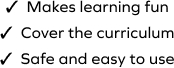# Missing Number to Subtract Doubles

SplashLearn - The complete PreK - Grade 5 Math & ELA Learning Program Built for Your Child
Home > Missing Number to Subtract Doubles
The game strengthens students' problem-solving ability while they develop fluency with the concepts of subtraction. In this task, students will use the previously learned strategy, that is, doubles to subtract strategy to solve the given problems. Students will drag and drop the item at the correct place to solve the problem.